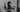# Making better decisions with the WADM

WADM is a formula to make mathematically better decisions based on factors that matter

Patrick Passarella - 7th Jan, 2021Photo by Piotr Makowski on Unsplash

WADM (Weighted Average Decision Matrix) is something I've learned to use that helped me make better professional and personal decisions. The first time I've heard of it was in the book The Millionaire Fastlane, since then, I have been using it sometimes. Usually, I don't like these types of "tools", but this one works well for me.

Making decisions is sometimes very hard, but important, and you also need to have confidence in it. There can be so much involved that it's better to have a structured way to think. When there are multiple alternatives to choose from, each alternative must be examined equally, or it could cost you the wrong decision consequences.

WADM objective is simple, make you decide between two or more things consistently, based on weighted factors that matter.

## How does it work

First, you'll need 3 things

• A list of criteria;
• A set of weights based on importance for the criteria;
• A set of alternatives.

Now with this information, it's easy to form a table, I will give an example. I want to decide what technology is best for me to learn. It's all fictional data since I don't have much experience in some of them. so don't take it personally.

CriteriaWeightReactAngularVue
Learn speed5425
Difficult2435
Community5543
Current Knowledge4353
Useful3532

### Criteria

These are the factors that I find most important for me to decide which technology I want to learn.

### Weight

The weight is what defines the criteria priority, I use 1 through 5, but you can use any format you like. 1 is the least important, and 5 the most important. A technology that has a strong community and the speed to learn is the top priority in this example.

### Alternatives

The options you want to compare. For me, it's three technologies, React, Angular, and Vue. And a rating for each factor on a number from 1 through 5. In this example, React got 5 in Community, because it has a great and active community, and Angular got 2 in Learn speed because I find it slower to learn.

Then, you multiply each option for each factor and add them together to get the total points for each alternative.

## How to apply WADM step by step

The WADM method is very flexible, but I find that there are ways that could help you get a more precise result. So I will try to write a general step by step process to use this formula whenever you need it.

1. List all the options you want to compare
eg: React, Angular, Vue.

2. List all the factors
eg: Learn speed, Difficult, Community, Current Knowledge, Useful.

3. Rate each option for each factor from 1 through 5
eg: 5 for React in Community, 5 for Vue in Learn speed.

4. Weight the factors from 1 through 5
eg: Community equals 5 weight, Difficult equals 2 weight.

We need to weigh each factor after rating the options, because doing it before may influence your choices and change your outcome.

5. Multiply each option point to the respective factor weight
eg: Difficult has weight 2, and React has a rating of 4 for the Difficult factor, so 4 * 2, which equals 8 points.

CriteriaWeightReactAngularVue
Learn speed54 * 5 = 202 * 5 = 105 * 5 = 25
Difficult24 * 2 = 83 * 2 = 65 * 2 = 10
Community55 * 5 = 254 * 5 = 203 * 5 = 15
Current Knowledge43 * 4 = 125 * 4 = 203 * 4 = 12
Useful35 * 3 = 153 * 3 = 92 * 3 = 6

6. Sum all the points

CriteriaWeightReactAngularVue
Learn speed5201025
Difficult28610
Community5252015
Current Knowledge4122012
Useful31596
Points-806570

In this fictional scenario, based on all the factors, React is a better choice. Maybe I overworked all of this, it's actually very straightforward. Give it a try! Whatever it is a personal or professional decision, it will help you choose more wisely.

Thanks for reading!Enjoyed the content or just want to send me a message? Follow me on Twitter!